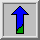# Pixel Subtraction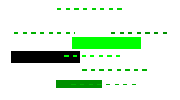Common Names: Pixel difference, Pixel subtract

The pixel subtraction operator takes two images as input and produces as output a third image whose pixel values are simply those of the first image minus the corresponding pixel values from the second image. It is also often possible to just use a single image as input and subtract a constant value from all the pixels. Some versions of the operator will just output the absolute difference between pixel values, rather than the straightforward signed output.

## How It Works

The subtraction of two images is performed straightforwardly in a single pass. The output pixel values are given by: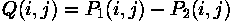Or if the operator computes absolute differences between the two input images then: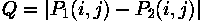Or if it is simply desired to subtract a constant value C from a single image then: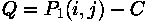If the pixel values in the input images are actually vectors rather than scalar values (e.g. for color images) then the individual components (e.g. red, blue and green components) are simply subtracted separately to produce the output value.

Implementations of the operator vary as to what they do if the output pixel values are negative. Some work with image formats that support negatively-valued pixels, in which case the negative values are fine (and the way in which they are displayed will be determined by the display colormap). If the image format does not support negative numbers then often such pixels are just set to zero (i.e. black typically). Alternatively, the operator may `wrap' negative values, so that for instance -30 appears in the output as 226 (assuming 8-bit pixel values).

If the operator calculates absolute differences and the two input images use the same pixel value type, then it is impossible for the output pixel values to be outside the range that may be represented by the input pixel type and so this problem does not arise. This is one good reason for using absolute differences.

## Guidelines for Use

Image subtraction is used both as a sub-step in complicated image processing sequences, and also as an important operator in its own right.

A common use is to subtract background variations in illumination from a scene so that the foreground objects in it may be more easily analyzed. For instance,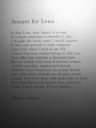shows some text which has been badly illuminated during capture so that there is a strong illumination gradient across the image. If we wish to separate out the foreground text from the background page, then the obvious method for black on white text is simply to threshold the image on the basis of intensity. However, simple thresholding fails here due to the illumination gradient. A typical failed attempt looks like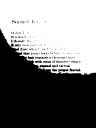Now it may be that we cannot adjust the illumination, but we can put different things in the scene. This is often the case with microscope imaging, for instance. So we replace the text with a sheet of white paper and without changing anything else we capture a new image, as shown inThis image is thelightfield. Now we can subtract the lightfield image from the original image to attempt to eliminate variation in the background intensity. Before doing that an offset of 100 is added to the first image to in order avoid getting negative numbers and we also use 32-bit integer pixel values to avoid overflow problems. The result of the subtraction is shown in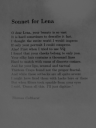Note that the background intensity of the image is much more uniform than before, although the contrast in the lower part of the image is still poor. Straightforward thresholding can now achieve better results than before, as shown in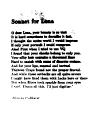which is the result of thresholding at a pixel value of 80. Note that the results are still not ideal, since in the poorly lit areas of the image the contrast (i.e. difference between foreground and background intensity) is much lower than in the brightly lit areas, making a suitable threshold difficult or impossible to find. Compare these results with the example described under pixel division.

Absolute image differencing is also used forchange detection. If the absolute difference between two frames of a sequence of images is formed, and there is nothing moving in the scene, then the output will mostly consist of zero value pixels. If however, there is movement going on, then pixels in regions of the image where the intensity changes spatially, will exhibit significant absolute differences between the two frames.

As an example of such change detection, considerwhich shows an image of a collection of screws and bolts. The imageshows a similar scene with one or two differences. If we calculate the absolute difference between the frames as shown in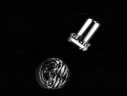then the regions that have changed become clear. The last image here has been contrast-stretched in order to improve clarity.

Subtraction can also be used to estimate the temporal derivative of intensity at each point in a sequence of images. Such information can be used, for instance, inoptical flow calculations.

Simple subtraction of a constant from an image can be used to darken an image, although scaling is normally a better way of doing this.

It is important to think about whether negative output pixel values can occur as a result of the subtraction, and how the software will treat pixels that do have negative values. An example of what may happen can be seen in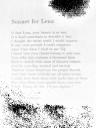which is the above lightfield directly subtracted from the text images. In the implementation of pixel subtraction which was used, negative values are wrapped around starting from the maximum value. Since we don't have exactly the same reflectance of the paper when taking the images of the lightfield and the text, the difference of pixels belonging to background is either slightly above or slightly below zero. Therefore the wrapping results in background pixels with either very small or very high values, thus making the image unsuitable for further processing (for example, thresholding). If we alternatively set all negative values to zero, the image would become completely black, because subtracting the pixels in the lightfield from the pixels representing characters in the text image yields negative results, as well.
In this application, a suitable way to deal with negative values is to use absolute differences, as can be seen in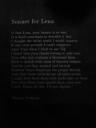or as a gamma corrected version in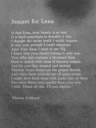Thresholding this image yields similar good results as the earlier example.

If negative values are to be avoided then it may be possible to first add an offset to the first input image. It is also often useful if possible to convert the pixel value type to something with a sufficiently large range to avoid overflow, e.g. 32-bit integers or floating point numbers.

## Interactive Experimentation

You can interactively experiment with this operator by clicking here.

## Exercises

1. Take images of your watch at two different times, without moving it in between, and use subtraction to highlight the difference in the display.
2. Use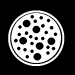to investigate the following method for edge detection. First apply erosion to the image and then subtract the result from the original. What is the difference in the edge image if you use dilation instead of erosion? What effects have size and form of the structuring element on the result. How does the technique perform on grayscale images?

## References

A. Jain Fundamentals of Digital Image Processing, Prentice Hall, 1989, pp 240 - 241.

R. Gonzales and R. Woods Digital Image Processing, Addison wesley, 1992, pp 47 - 51, 185 - 187.

R. Boyle and R. Thomas Computer Vision: A First Course, Blackwell Scientific Publications, 1988, p 35.

A. Marion An Introduction to Image Processing, Chapman and Hall, 1991, pp 238 - 241.

D. Vernon Machine Vision, Prentice-Hall, 1991, pp 52 - 53.

## Local Information